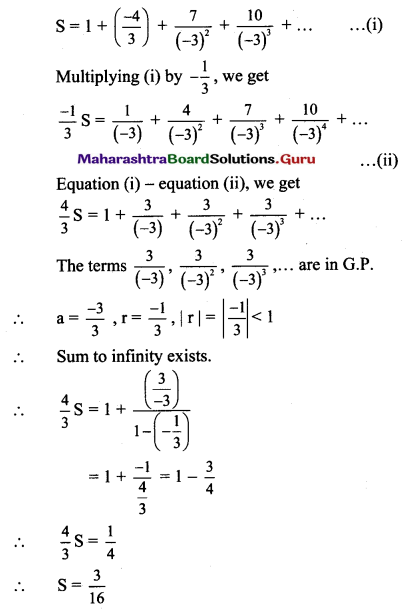Balbharti Maharashtra State Board 11th Maths Book Solutions Pdf Chapter 2 Sequences and Series Ex 2.5 Questions and Answers.

## Maharashtra State Board 11th Maths Solutions Chapter 2 Sequences and Series Ex 2.5

Question 1.
Find Sn of the following arithmetico-geometric sequences.
(i) 2, 4x, 6x2, 8x3, 10x4, ……
Solution:
2, 4x, 6x2, 8x3, 10x4, ……
Here, 2, 4, 6, 8, 10,… are in A.P.
∴ a = 2, d = 2
∴ nth term = a + (n – 1)d
= 2 + (n – 1)(2)
= 2n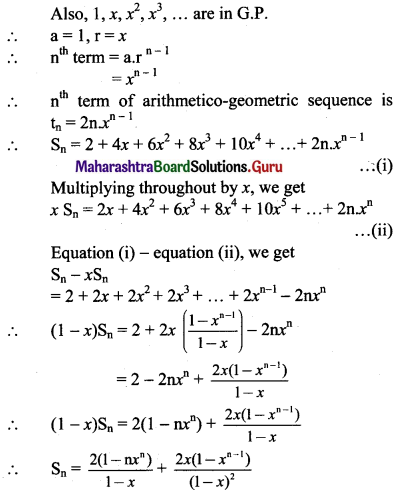(ii) 1, 4x, 7x2, 10x3, 13x4, ……
Solution:
1, 4x, 7x2, 10x3, 13x4, ……
Here, 1, 4, 7, 10, 13,… are in A.P.
a = 1, d = 3
∴ nth term = a + (n – 1)d
= 1 + (n – 1)(3)
= 3n – 2
Also, 1, x, x2, x3,… are in G.P.
∴ a = 1, r = x,
nth term = arn-1 = xn-1
nth term of arithmetico-geometric sequence is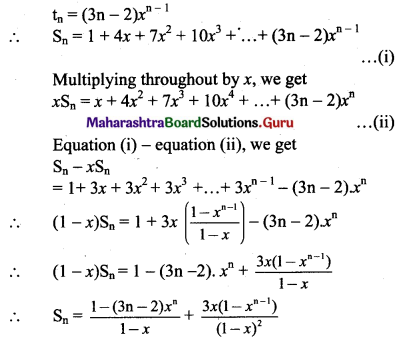(iii) 1, 2 × 3, 3 × 9, 4 × 27, 5 × 81, ……
Solution:
1, 2 × 3, 3 × 9, 4 × 27, 5 × 81, …..
Here, 1, 2, 3, 4, 5, … are in A.P.
∴ a = 1, d = 1
∴ nth term = a + (n – 1)d
= 1 + (n – 1)1
= n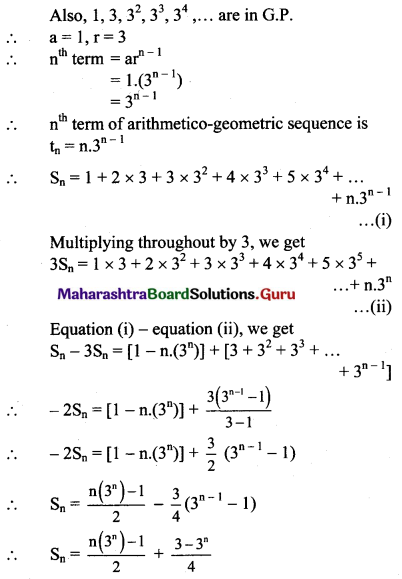(iv) 3, 12, 36, 96, 240, ……
Solution:
3, 12, 36, 96, 240, ……
i.e., 1 × 3, 2 × 6, 3 × 12, 4 × 24, 5 × 48, …….
Here, 1, 2, 3, 4, 5, ….. are in A.P.
∴ nth term = n
Also, 3, 6, 12, 24, 48, ….. are in G.P.
∴ a = 3, r = 2
∴ nth term = arn-1 = 3 . (2n-1)
∴ nth term of arithmetico-geometric sequence is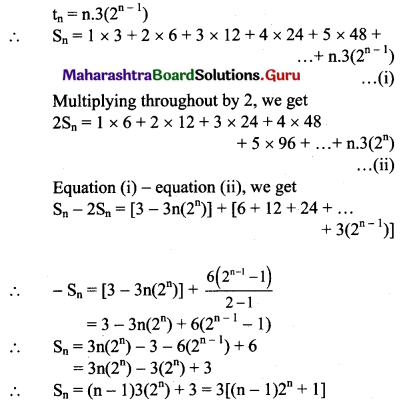Question 2.
Find the sum to infinity of the following arithmetico-geometric sequence.
(i) $$1, \frac{2}{4}, \frac{3}{16}, \frac{4}{64}, \ldots$$
Solution: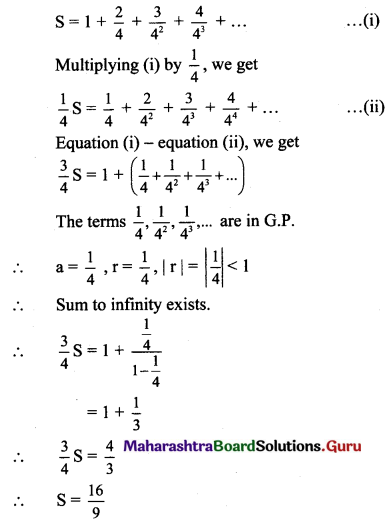(ii) $$3, \frac{6}{5}, \frac{9}{25}, \frac{12}{125}, \frac{15}{625}, \ldots$$
Solution: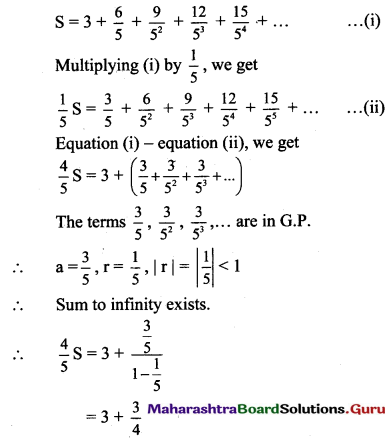∴ $$\frac{4}{5} S=\frac{15}{4}$$
∴ S = $$\frac{75}{16}$$

(iii) $$1, \frac{-4}{3}, \frac{7}{9}, \frac{-10}{27} \ldots$$
Solution: